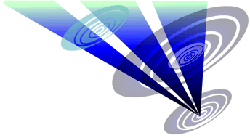Reconstruction of the 2015 Foxley Road formation 1.Draw a circle. Draw and extend the horizontal and vertical centerlines. 2.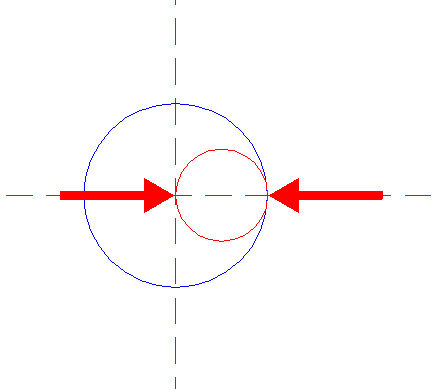Construct a "two-points" circle (defined by the two end-points of a centerline) between the center of circle 1 and its righthand intersection with the horizontal centerline. 3.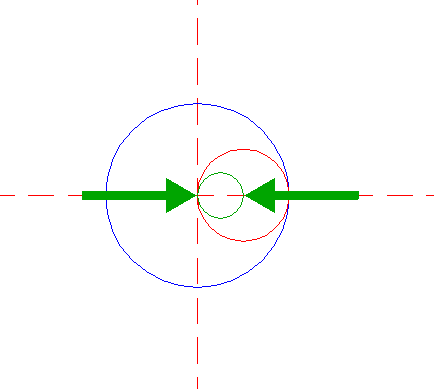Construct a "two-points" circle between the centers of circles 1 and 2. 4.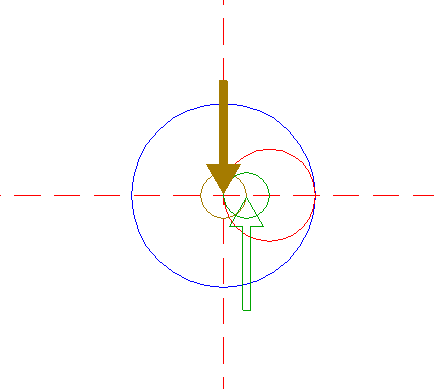Copy circle 3 to the center of circle 1. 5.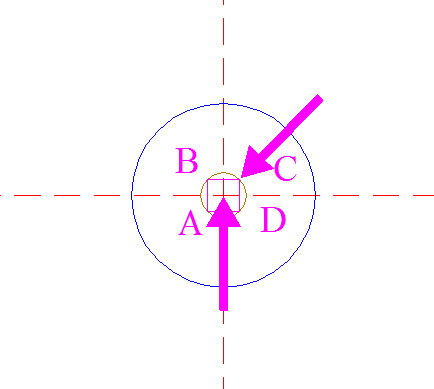Construct the inscribed square of circle 4, with horizontal and vertical sides. Call the angular points A, B, C, and D, clockwise, starting at the lower lefthand one. 6.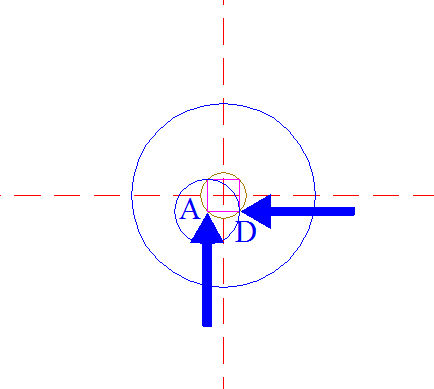Construct a circle centered at point A, passing through point D. 7.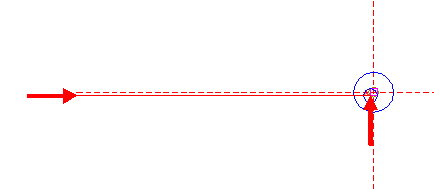Draw a line from point A parallel to the horizontal centerline, extending to the left. See detail.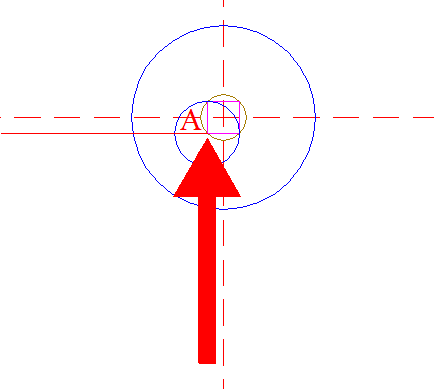8.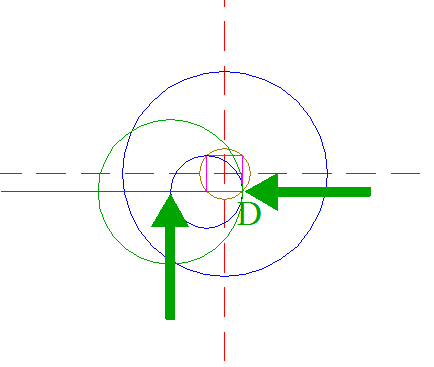Construct a circle centered at the intersection of circle 6 and line 7, passing through point D. 9.Copy circle 8 to the lower intersection of square 5 and the vertical centerline. 10.Copy circle 9 twenty times, the first to its lefthand intersection with line 7, the others consecutively to their corresponding intersections, as shown. See detail.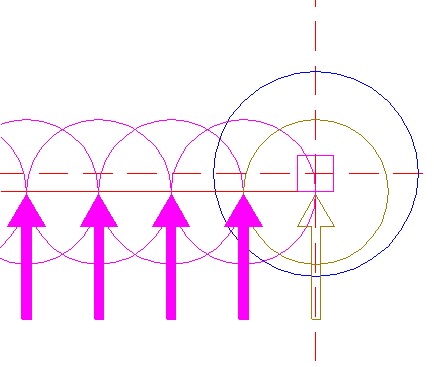11.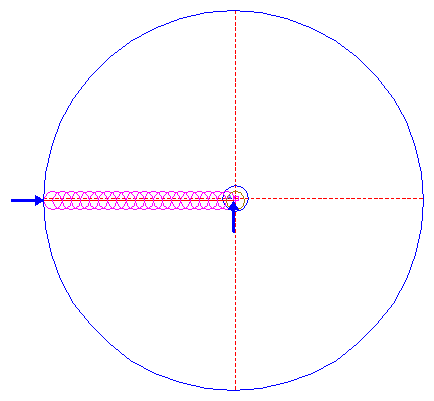Construct a circle centered at point A, tangent to the leftmost circle 10 at the lefthand side. See detail.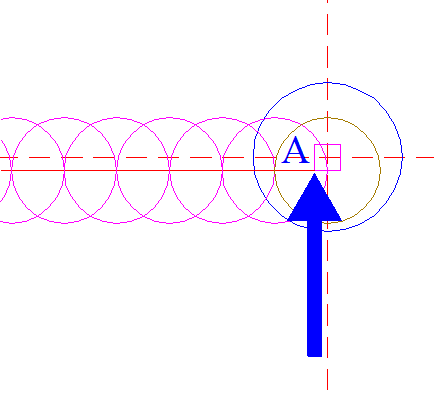12.Draw a line from point B parallel to the vertical centerline, extending upward. See detail.13.Construct twenty arcs, centered at point A, from the lefthand intersections of circles 10 and line 7 upto line 12, clockwise, as shown. 14.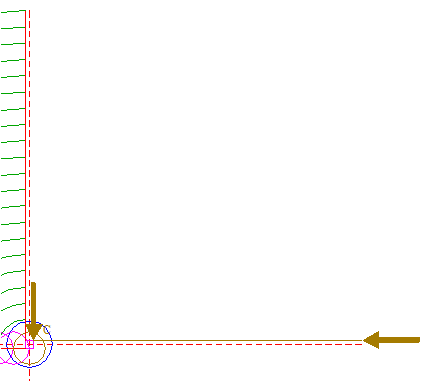Draw a line from point C parallel to the horizontal centerline, extending to the right. 15.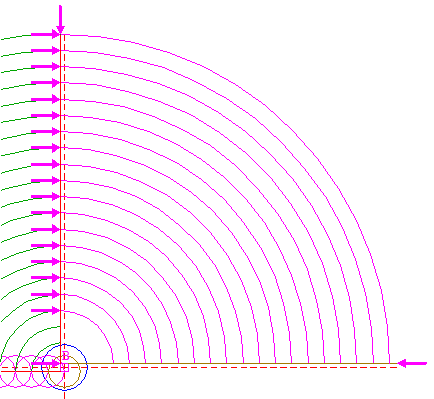Construct eighteen arcs, centered at point B, from the intersections of arcs 13, except for the two innermost arcs, and line 12 upto line 14, clockwise. 16.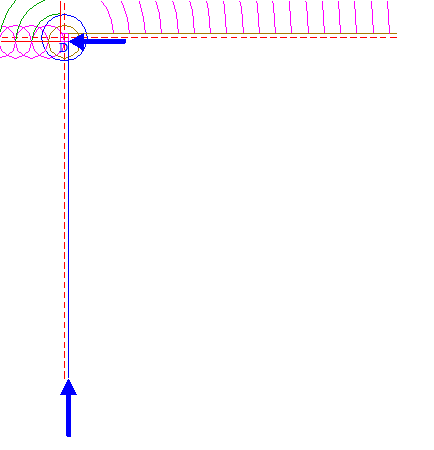Draw a line from point D parallel to the vertical centerline, extending downward. 17.Construct eighteen arcs, centered at point C, from the intersections of arcs 15 and line 14 upto line 16, clockwise. 18.Construct eighteen arcs, centered at point D, from the intersections of arcs 17 and line 16 upto line 7, clockwise.Notice, that arcs 13, 15, 17, and 18, are a piecewise approximation of a real spiral! 19.Construct a circle centered at the intersection of circle 1 and line 12, passing through the intersection of the second smallest arc 13 and line 12. 20.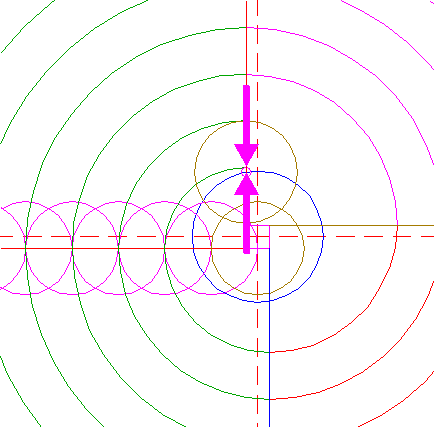Construct a circle concentric to circle 19, passing through the intersection of the smallest arc 13 and line 12. 21.Construct the inscribed regular 20-sided polygon of circle 11, pointing up. 22.Draw a line from point A to the lefthand angular point of polygon 21, just above the horizontal centerline. See detail.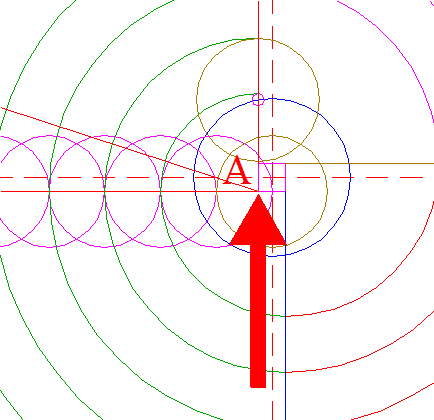23.Circles 1, 19, and 20, arcs 13, 15, 17, and 18, and line 22 are used for the final reconstruction. 24.Remove all parts not visible within the formation itself. 25.Colour all areas corresponding to standing... 26....or to flattened crop, and finish the reconstruction of the 2015 Foxley Road formation. 27.The final result, matched with the aerial image.